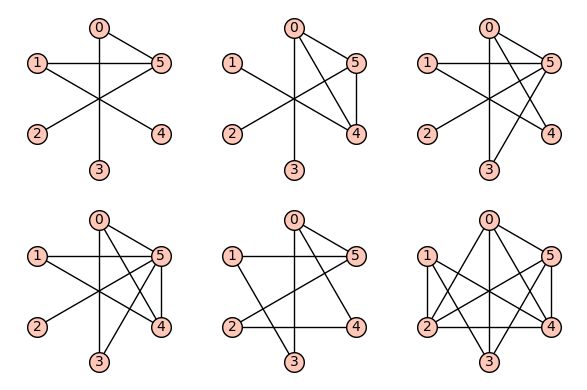Ask Your Question

# Consider the class of all possible connected simple graphs on $n$ verticesAnonymous

Consider the class of all possible connected simple graphs on $n$ vertices ($n$ is any natural number, we can choose any natural number). Now from this collection, can we find those graphs (if there exists any) satisfying the following property: Suppose that $A$ denotes the usual $(0,1)$ adjacency matrix of a graph. Now can we find those graphs explicitly (if there is any) on $n$ vertices such that if $\lambda$ is an eigenvalue of $A$, then $\dfrac{1}{\lambda}$ is also an eigenvalue and if $\alpha$ is another eigenvalue (distinct from $\lambda$), then $-\dfrac{1}{\alpha}$ is an eigenvalue. Basically eigenvalues of $A$ are of the form $(\lambda,\dfrac{1}{\lambda})$ and $(\alpha,-\dfrac{1}{\alpha})$.

Basically I am trying to find the graphs for which some roots of the form $(\lambda,\dfrac{1}{\lambda})$, and some roots of the form $(\alpha,-\dfrac{1}{\alpha})$.

please help regarding this problem

edit retag close merge delete

## Comments

This seems very related to Ask Sage question 54878.

The condition is now relaxed from "for any eigenvalue, both its inverse and the opposite of its inverse are also eigenvalues" to "for any eigenvalue, either its inverse or the opposite of its inverse is also an eigenvalue". Is that right?

## 1 Answer

Sort by » oldest newest most voted

We adapt the method provided in an answer to Ask Sage question 54878 and define a function that provides an iterator over graphs on n vertices with the requested property.

We then treat the case of n = 6 as an example, and plot all the corresponding graphs.

Function:

def mygraphs(n):
return (g for g in graphs.nauty_geng(f"{n} -c")
if all(e and (1/e in ee or -1/e in ee)
for ee in [g.adjacency_matrix().eigenvalues()]
for e in ee))


List of graphs on 6 vertices with the requested property

sage: G6 = list(mygraphs(6))
sage: len(G6)
6


View them:

sage: opt = {'axes': False, 'aspect_ratio': 1, 'color': 'grey', 'alpha': 0}
sage: p = point2d([(-1.2, -1.2), (1.2, 1.2)], **opt)
sage: gg = [p + g.plot(layout='circular') for g in G6]
sage: graphics_array(gg, ncols=3)more

## Your Answer

Please start posting anonymously - your entry will be published after you log in or create a new account.

Add Answer

## Stats

Asked: 2020-12-26 16:57:22 +0200

Seen: 64 times

Last updated: Dec 27 '20